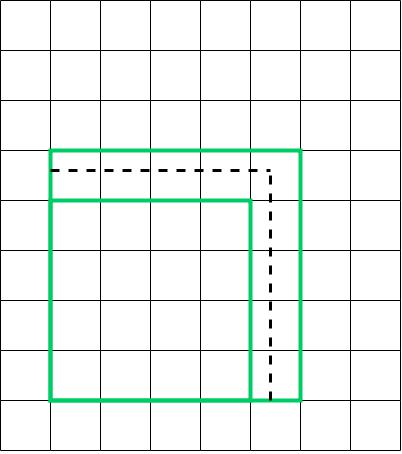# Determine square roots of rational numbers#### All in One Place

Everything you need for better grades in university, high school and elementary.#### Learn with Ease

Made in Canada with help for all provincial curriculums, so you can study in confidence.#### Instant and Unlimited Help

0/15
##### Examples
###### Lessons
1. Use the graph below to determine a rational number with a square root between 4 and 5.1. Use the side lengths below to estimate and calculate the area of each square.
1. 5.2 cm
2. 0.027 km
2. Find out if each of the following rational numbers is a perfect square.
1. $\frac{{81}}{{16}}$
2. 0.1
3. 0.01
4. $\frac{5}{{14}}$
3. Evaluate.
1. $\sqrt {361}$
2. $\sqrt {2209}$
3. $\sqrt {0.0169}$
4. $\sqrt {5.76}$
4. Calculate.
1. $\sqrt {56}$, to the nearest tenth
2. $\sqrt {3.7}$, to the nearest hundredth
3. $\sqrt {0.96}$ , to the nearest hundredth
4. $\sqrt {0.066}$ , to the nearest hundredth
0%
##### Practice
###### Topic Notes
In this section, we will look at how to evaluate a rational number by using square roots. We will also work on questions determining whether a rational number is a perfect square. We will also evaluate the square roots of rational numbers.